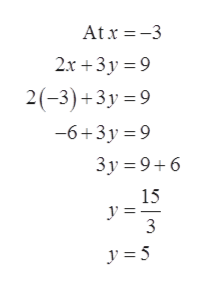# Consider the following function. 2x+3y=9Use the domain {-3,0,6} to find solution points that should be plotted when graphing this function. (Select all that apply.) (6,-1)(6,-3)(-3,5)(0,4)(-3,7)(0,3)

Question
9 views

Consider the following function.

2x+3y=9

Use the domain {-3,0,6} to find solution points that should be plotted when graphing this function. (Select all that apply.)

(6,-1)

(6,-3)

(-3,5)

(0,4)

(-3,7)

(0,3)

check_circle

Step 1

To determine the solution of the equation 2x + 3y = 9 using the domain {-3, 0, 6} and select the points from the following list (6,-1), (6,-3), (-3,5), (0,4), (-3,7), (0,3) needed to graph the equation 2x + 3y = 9.

Step 2

Consider the equation 2x + 3y = 9 and the domain {-3, 0, 6}.

Substitute -3 for x in the equation 2x + 3y = 9 and determine the value of y as given below:help_outlineImage TranscriptioncloseAtx -3 2x +3y 9 2(-3)+3y 9 -6+3y 9 3y 96 15 y=_ 3 fullscreen
Step 3

Substitute 0 for x in the equation 2x + 3y = 9 and determine ...

### Want to see the full answer?

See Solution

#### Want to see this answer and more?

Solutions are written by subject experts who are available 24/7. Questions are typically answered within 1 hour.*

See Solution
*Response times may vary by subject and question.
Tagged in

### Algebra